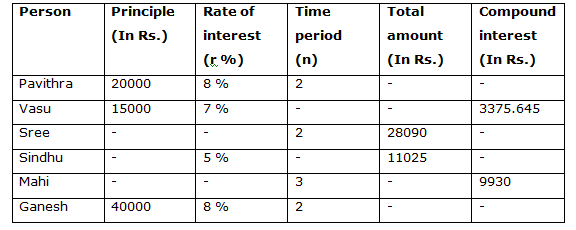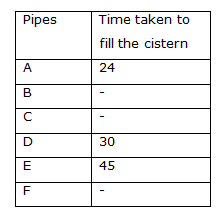# Quantitative Aptitude Questions (Data Interpretation) for RRB Assistant Mains 2018 Day-179

Dear Readers, IBPS RRB is conducting Online Examination for the recruitment of Assistant. To enrich your preparation here we have providing new series of Data Interpretation – Quantitative Aptitude Questions. Candidates those who are appearing in RRB Assistant  Mains Exams can practice these Quantitative Aptitude average questions daily and make your preparation effective.

[WpProQuiz 3417]

Click “Start Quiz” to attend these Questions and view Solutions

Directions (1 – 5): Study the following information carefully and answer the given questions:

The table below shows the sum invested by different person, rate of interest, time period, total amount they received and the compound interest they received at the end. Some data’s are missing.1) Find the total amount invested by Pavithra, if the interest compounded annually?

a) Rs. 24136

b) Rs. 23782

c) Rs. 23328

d) Rs. 22994

e) None of these

2) If the ratio between the principle amount invested by Vasu and Sree is 3 : 5, then find the rate of interest for Sree(if the interest compounded annually)?

a) 8 %

b) 10 %

c) 5 %

d) 6 %

e) None of these

3) If the total principle amount invested by Sindhu is half of the principle amount invested by Pavithra, then find the number of years the principle amount invested by Sindhu (if the interest compounded annually)?

a) 2 years

b) 1 year

c) 3 years

d) 4 years

e) None of these

4) Find the average principle amount invested by Pavithra, Vasu, Mahi and Ganesh, if the ratio of rate of interest for Sindhu and Mahi is 1: 2 (if the interest compounded annually)?

a) Rs. 25780

b) Rs. 26250

c) Rs. 26600

d) Rs. 27125

e) None of these

5) Find the compound interest received by Ganesh, if the interest compounded half yearly?

a) Rs. 5987.128

b) Rs. 6523.276

c) Rs. 6652.784

d) Rs. 6794.342

e) None of these

Directions (6 – 10): Study the following information carefully and answer the given questions:

The following table represents time taken (in hours) by different pipes to fill a cistern. Some values are missing.6) If A and C kept open for 4 hours then A is replaced by D and kept open for 5 more hours, the tank is filled. In how many hours pipe C alone can fill the cistern?

a) 7 ¾ hours

b) 13 ½ hours

c) 6 5/6 hours

d) 12 ¾ hours

e) None of these

7) Two pipes D and E are opened simultaneously to fill the cistern. After how much time should D be closed so that E alone can fill the cistern in another 20 hours?

a) 8 hours

b) 14 hours

c) 12 hours

d) 10 hours

e) None of these

8) If C takes half of the time taken by F to fill the cistern and F takes half of the time taken by B to fill the cistern and all of them working together can fill the cistern in 48 hours, What is the time taken by F to fill the cistern?

a) 152 hours

b) 144 hours

c) 186 hours

d) 168 hours

e) None of these

9) Two pipes A and D can fill the cistern. If they are opened on alternative hours and if pipe A is opened first, in how many hours will the cistern be full?

a) 24 (1/3) hours

b) 26 (3/5) hours

c) 26 (3/4) hours

d) 25 (1/2) hours

e) None of these

10) Three pipes A, D and F together can fill the cistern in 8 hours. Find the time taken by F alone to fill the cistern?

a) 20 hours

b) 15 hours

c) 18 hours

d) 12 hours

e) None of these

Direction (1-5) :

P = Rs. 20000, r = 8 %, n = 2 years

Compound interest:

20000*(8/100) = 1600

21600*(8/100) = 1728

C.I = 1600 + 1728 = Rs. 3328

Total amount invested by Pavithra = 20000 + 3328 = Rs. 23328

The principle amount invested by Sree = 15000*(5/3) = Rs. 25000

Total amount received by Sree = Rs.28090

Time period (n) = 4 years

Amount = P*(1 + (r/100))n

28090= 25000*((100 + r)/100)2

(2809/2500) = ((100 + r)/100)2

(53/50) = (100 + r)/100

106 = 100 + r

Rate of interest (r) = 6 %

The total principle amount invested by Sindhu = (1/2)*20000 = Rs. 10000

Rate of interest (r) = 5 %

Total amount received by Sindhu = Rs. 11025

Amount = P*(1 + (r/100))n

11025 = 10000*((100 + 5)/100)n

11025/10000 = (21/20)n

441/400 = (21/20)n

(21/20)2 = (21/20)n

Number of years (n) = 2 years

Rate of interest for Mahi = (5/1)*2 = 10 %

Time period (n) = 3 years, C.I = Rs. 9930

C.I = P*[(1 + (r/100))n – 1]

9930 = P*[(110/100)3 – 1]

9930 = P*[(11/10)3 – 1]

9930 = P*[(1331/1000) – 1]

9930 = P*[331/1000]

P = 9930*(1000/331) = Rs. 30000

Required average = (20000 + 15000 + 30000 + 40000)/4 = 105000/4

= > Rs. 26250

Total amount = 40000*(104/100)*(104/100)*(104/100)*(104/100)

= > 46794.342

Compound interest = 46794.342 – 40000 = Rs. 6794.342

Direction (6-10) :

Let C takes x hours to fill the cistern,

According to the question:

4/24 + 4/x + 5/x + 5/30 = 1

=> 1/6 + 9/x + 1/6 = 1

=> 2/6 + 9/x = 1

=> 1/3 + 9/x = 1

=> 9/x = 1 – (1/3)

=> 9/x = 2/3

=> x = 27/2 = 13 ½ hours

C alone can fill the cistern in 13 ½ hours

Let D should be closed after x hours,

x/30 + x/45 + 20/45 = 1

=> (3x + 2x)/90 = 1 – (4/9)

=> 5x/90 = 5/9

=> x = 10 hours

D should be closed after 10 hours

C takes half of the time taken by F to fill the cistern

= > C : F = 1 : 2

F takes half of the time taken by B to fill the cistern

= > F : B = 1 : 2

The ratio of C, F and B taken to fill the cistern = 1 : 2 : 4 (x, 2x, 4x)

According to the question:

1/x + 1/2x + 1/4x = 1/48

= > (4 + 2 + 1)/4x = 1/48

= > 7/4x = 1/48

= > 4x = 48*7

= > x = 84 hours

Time taken by F to fill the cistern = 2*84 = 168 hours

Part of the tank filled in 2 hours = 1/24 + 1/30 = 3/40

= > Part of the tank filled in 26 hours = (3/40)*13 = 39/40

Remaining part = 1 – 39/40 = 1/40

Time taken by A to fill the remaining part,

= > (1/40)*24 = 3/5

Total time = 26 + 3/5 = 26 (3/5) hours

Let the time taken by F to fill the cistern be x,

According to the question,

(1/24) + (1/30) + (1/x) = (1/8)

(1/x) = (1/8) – [(1/24) + 1/30)]

(1/x) = (1/8) – (3/40)

(1/x) = 2/40

1/x = 1/20

F alone to fill the cistern is 20 hours

Daily Practice Test Schedule | Good Luck

 Topic Daily Publishing Time Daily News Papers & Editorials 8.00 AM Current Affairs Quiz 9.00 AM Quantitative Aptitude “20-20” 11.00 AM Vocabulary (Based on The Hindu) 12.00 PM General Awareness “20-20” 1.00 PM English Language “20-20” 2.00 PM Reasoning Puzzles & Seating 4.00 PM Daily Current Affairs Updates 5.00 PM Data Interpretation / Application Sums (Topic Wise) 6.00 PM Reasoning Ability “20-20” 7.00 PM English Language (New Pattern Questions) 8.00 PM